4.4 Analysis of Skiplists

In this section, we analyze the expected height, size, and length of the search path in a skiplist. This section requires a background in basic probability. Several proofs are based on the following basic observation about coin tosses.

Lemma 4..2   Letbe the number of times a fair coin is tossed up to and including the first time the coin comes up heads. Then.

Proof. Suppose we stop tossing the coin the first time it comes up heads. Define the indicator variableNote that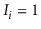if and only if the firstcoin tosses are tails, so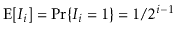. Observe that, the total number of coin tosses, can be written as. Therefore,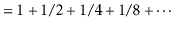The next two lemmata tell us that skiplists have linear size:

Lemma 4..3   The expected number of nodes in a skiplist containing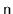elements, not including occurrences of the sentinel, is.

Proof. The probability that any particular element,, is included in list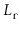is, so the expected number of nodes inis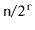.4.2 Therefore, the total expected number of nodes in all lists is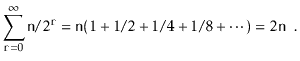Lemma 4..4   The expected height of a skiplist containingelements is at most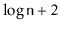.

Proof. For each, define the indicator random variable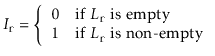The height,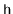, of the skiplist is then given byNote that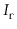is never more than the length,, of, soTherefore, we have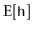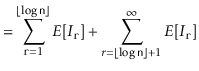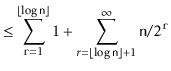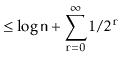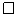Lemma 4..5   The expected number of nodes in a skiplist containingelements, including all occurrences of the sentinel, is.

Proof. By Lemma 4.3, the expected number of nodes, not including the sentinel, is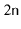. The number of occurrences of the sentinel is equal to the height,, of the skiplist so, by Lemma 4.4 the expected number of occurrences of the sentinel is at most.Lemma 4..6   The expected length of a search path in a skiplist is at most.

Proof. The easiest way to see this is to consider the reverse search path for a node,. This path starts at the predecessor ofin. At any point in time, if the path can go up a level, then it does. If it cannot go up a level then it goes left. Thinking about this for a few moments will convince us that the reverse search path foris identical to the search path for, except that it is reversed.

The number of nodes that the reverse search path visits at a particular level,, is related to the following experiment: Toss a coin. If the coin comes up as heads, then move up and stop. Otherwise, move left and repeat the experiment. The number of coin tosses before the heads represents the number of steps to the left that a reverse search path takes at a particular level.4.3 Lemma 4.2 tells us that the expected number of coin tosses before the first heads is 1.

Letdenote the number of steps the forward search path takes at levelthat go to the right. We have just argued that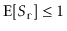. Furthermore,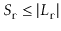, since we can't take more steps inthan the length of, so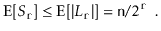We can now finish as in the proof of Lemma 4.4. Let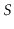be the length of the search path for some node,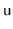, in a skiplist, and letbe the height of the skiplist. Then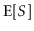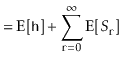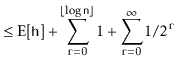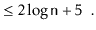The following theorem summarizes the results in this section:

Theorem 4..3   A skiplist containingelements has expected size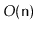and the expected length of the search path for any particular element is at most.

Footnotes

....4.2
See Section 1.3.4 to see how this is derived using indicator variables and linearity of expectation.
... level.4.3
Note that this might overcount the number of steps to the left, since the experiment should end either at the first heads or when the search path reaches the sentinel, whichever comes first. This is not a problem since the lemma is only stating an upper bound.
opendatastructures.org